#### How to Calculate Bmi in Excel

The calculation of BMI (Body Mass Index) is purposely meant to know if the person has a normal, overweight, or obese weight. This is possibly done by certain components that make the BMI calculation a possibility. We will create the calculator together, and even calculate it.

## What is BMI?

BMI equation is:

BMI = weight (kg) / height (m)2

or easier:

BMI = kg/m2

In this formula, the weight is the weight of the person in kilograms, and the height is the height of the person in meters. The resulting value is the BMI.

The BMI test is one of the basic methods used by nutritionists, doctors and personal trainers to assess the body shape in terms of weight.

### Simplified / Basic Classification of BMI Results

• <18.5 – underweight
• 18.5–24.99 – correct value, i.e. normal
• ≥ 25.0 – overweight

Or more specifically:

• <16.0 – starvation
• 16.0–16.99 – emaciation
• 17.0–18.49 – underweight
• 18.5–24.99 – correct value
• 25.0–29.99 – overweight
• 30.0–34.99 – 1st degree of obesity
• 35.0-39.99 – II degree of obesity (clinical obesity)
• ≥ 40.0 – III degree of obesity (extreme obesity); exceeding this threshold is also called morbid obesity, which is a serious threat to health

These ranges are based on research that has shown a correlation between BMI and the risk of health problems such as heart disease, high blood pressure, and diabetes.

## Data preparation

Display the weight (1) and height (2) of the person in kilograms and meters.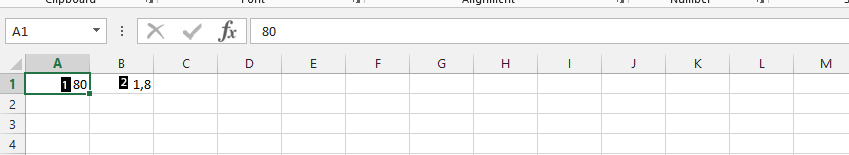For example, in cell C1, click on it (1), divide the weight by height, and add ^2 (2), and press enter.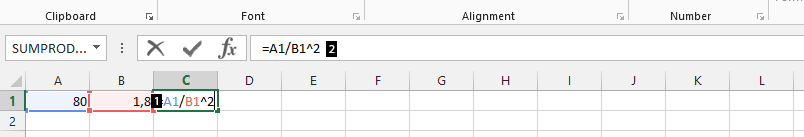## BMI Calculator

To categorize the BMI values into these ranges in Excel, you can use the IF function. The IF function allows you to specify a set of conditions, and then return a different value depending on which condition is met. In the case of BMI, you can use the IF function to return a text value such as “Underweight”, “Normal weight”, “Overweight”, or “Obese”, depending on the BMI value calculated.

Use if function to calculate a bmi in Excel.

Click on another empty cell (D1) (1), and type =IF(AND(C1>=18.5,C1<=25),”Normal”) (2).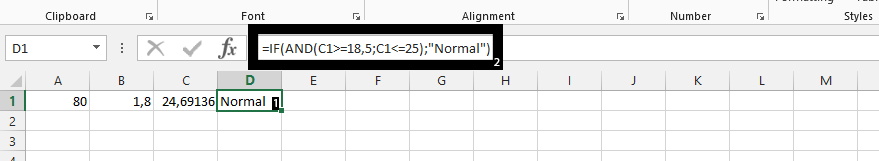Click on another empty cell (E1) (1), and type in what is shown in 2 in the picture below.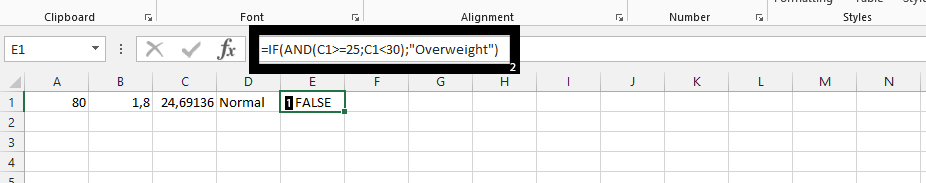Click on another empty cell (1), and type in number 2.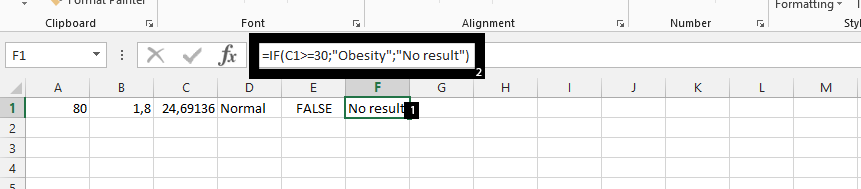In conclusion, if it happens that the person is below 18.5, then such a person is underweight, and the 80 is in kilograms, while 1.8 is in meters, which means the person is 180 cm in height.

You already know what BMI is, what BMI stands for, how to calculate BMI and how to interpret BMI results.